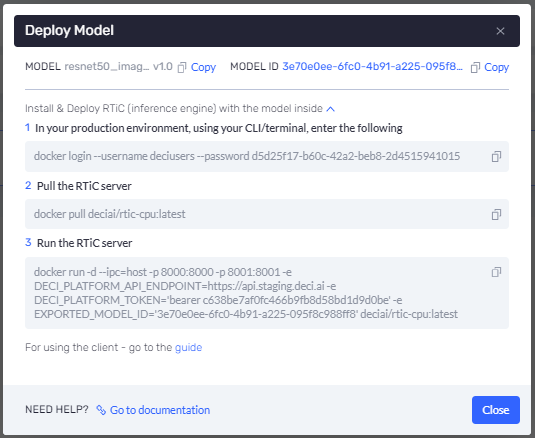# Measuring the Model's Performance

To measure a model’s performance –

(1) Run the client.rtic.measure command from your application, as follows –

``````client.rtic.measure(model_name='Your_Model_Name',
batch_size=8,
repetitions=5,
input_dims=(3,224,224))
``````

The request consists of the following parameters –

• Your_Model_Name must exactly match the name of the model being measured. This name appears in the top left corner of the Deploy Model window, as shown below –• BATCH_SIZE is the batch size for which the measurement will be made. This should be the batch size that the model is configured to handle.
• REPETITIONS is the number of times the measurement request will be sent in order to improve accuracy. The measurement that is presented in the Deci platform represents the average of the measurements in the responses to each of these requests.
• INPUT_DIMS is the size/shape of the input to be used to measure the model’s performance. This should be the size/shape that the model is configured to handle.

The following is an example of a request –

``````YOUR_MODEL_NAME = 'ResNet_50_1_0'

client.rtic.measure(model_name=YOUR_MODEL_NAME,
batch_size=8,
input_dims=(3,224,224))
``````

The following is an example of a response –

``````>>> {'data': {'batch_inf_time': 133.015214073181152,
'batch_size': 8,
'memory': 0.4,
'sample_inf_time': 29.178214073181152,
'throughput': 34.272145563533286},
'message': 'Successfully measured the model',
'success': True}
``````

The following describes the response parameters –
'batch_inf_time' – Specifies the latency for the entire batch.
'sample_inf_time' – Specifies the latency for a specific request.
'memory' – Specifies the memory footprint that the model utilizes while inferencing.
'throughput' – Specifies the quantity of requests handled per milli-second.

You may refer to the RTiC API reference documentation for more information.